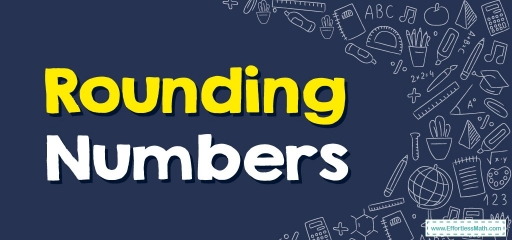# Rounding Numbers## What is Rounding Numbers?

Rounding a number is the procedure of making the number simpler so the value stays near what it was. The outcome you get after you round off a number is not as accurate, however, it’s simpler to use.

When rounding numbers, we take into consideration the digit’s place value in the number. You can round numbers to different digits, such as, you can round one to the closest ten, hundred, thousand, etc.

Rounding is frequently performed to get a value that is simpler to report and send out than the former. Rounding might additionally be crucial to avoiding misleadingly precise commentary of a calculated number, estimate, or measurement.

Note: Calculations completed via rounding ought to be near the ones completed with no rounding.

## Rounding numbers to the closest 10

• Rounding a number to the closest ten implies we must check the digit sitting to the right of the tens position, which is the ones position.

## Rounding a number to the closest 100

• Rounding a number to the closest hundred implies we must check the digit sitting to the right of the hundreds position, which is the tens position.

## Rounding-Up and Rounding-Down

As rounding is a generic word, we generally apply the phrases, ’rounding up’ or ’rounding down’ to indicate if the number is bigger or smaller after it’s rounded. Whenever a rounded number is raised, the provided number has been rounded up, while, if a rounded number is reduced, it has been rounded down.

## Guidelines for Rounding a Number

How do people choose which value is more applicable in-between different estimated values of one number? Ought we pick one bigger than the original or decide to use a smaller one?

Whenever one “rounds to the nearest _____” no matter what you put into the blank you pretty much always do the same steps:

Step one: Firstly you must know which one is the digit to round. That number is the digit ultimately affected.

Step two: Then, we must check the number sitting at the right of that position and this determines what happens to the rounding number.

Step three: If the number on the right is lower than five, don’t alter the rounding digit. But, every digit to the right of that rounding digit gets altered to zero.

Step four: If the digit on the right is given or higher, you raise the rounding digit by $$1$$, as well as change all the digits on the right of that rounding digit to zero.

### Rounding Numbers – Example 1:

You would round $$8312$$ to the closest hundred.

• Step one: Change the place value up to whatever that number should be rounded to. Therefore, $$8312$$ must get rounded to the closest hundred. Hence, you mark $$3$$ as that’s in the hundreds spot.
• Step two: Watch and underline the number on the right of $$3$$, as it’s in the tens position. So, it’s $$1$$, and you’d mark that: $$83$$1$$2$$
• Step three: Do a comparison of the digit that’s underlined with $$5$$.
• Step four: If it’s lower than $$5$$, every digit to the right along with should get replaced by zero and the number in the hundreds position $$(3)$$ isn’t altered. So, $$8312$$ gets rounded down to $$8300$$.

Note: If a number on the right of the $$3$$ was $$5$$ or more, then every digit on the right of $$3$$ ends up being $$0$$, and $$3$$ is going to raise by $$1$$, thus becoming $$4$$. For instance, if the provided digit was $$3176$$, it is going to get rounded upward to $$4200$$ (to the closest hundred).

### Rounding Numbers – Example 2:

Round each number to the nearest hundred.

$$572$$

Solution:

First, look at the next place value to the right (hundred). It’s $$7$$ and it is greater than $$5$$. Thus, add $$1$$ to the digit in the hundreds place. The answer is $$600$$

## Exercises for Rounding Numbers

Round each number to the nearest thousand.

1. $$\color{blue}{7,152}$$
2. $$\color{blue}{5,563}$$
3. $$\color{blue}{1,734}$$
4. $$\color{blue}{4,351}$$
1. $$\color{blue}{7,000}$$
2. $$\color{blue}{6,000}$$
3. $$\color{blue}{2,000}$$
4. $$\color{blue}{4,000}$$

### What people say about "Rounding Numbers - Effortless Math: We Help Students Learn to LOVE Mathematics"?

No one replied yet.

X
30% OFF

Limited time only!

Save Over 30%

SAVE $5 It was$16.99 now it is \$11.99